## 2.3.8 Victora method2.5

In the cases where neither the energy barrier nor the attempt frequency is a function of the temperature the Arrhenius-Néel law is a simple relation between temperature and the time elapsed in the considered thermally activated process. Larger temperature corresponds to smaller elapsed time and the inverse also holds true. However, in the Arrhenius-Néel law there are two factors that are not easy to calculate, the attempt frequency and the energy barrier. In the axially symmetric case the attempt frequency is temperature dependent, but in other less simple cases the prefactor will be weakly dependent on temperature. If this happens, we can use a set of four equations to eliminate the prefactor and the energy barrier value from the problem. Based on that, J. Xue et al. [Xue 00] proposed a method to obtain simulation for larger times, not achievable numerically, calculating only small time intervals at higher temperatures. The method is applied to the calculation of the hysteresis loops and, therefore, it is more convenient to express the time scales as the inverse of the applied field sweep rate.

The method is as follows: we simulate a loop at a reference temperature and at a reference sweep rate, being this reference sweep rate as slow as feasible. Next we try to find the temperature of the fast sweep rate loop that matches the reference loop. The different variables will be related by the formula(2.63)

where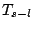is the temperature to perform the equivalent loop,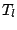the temperature for the large time scale,the reference temperature,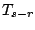the temperature obtained from the matching of the loops,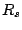the small time scale sweep rate,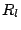the large time scale sweep rate and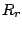the reference sweep rate. Finally, we calculate a loop at high temperatureand fast sweep rate. The result of this last simulation is equivalent, according to the method, to the direct calculation of the desired loop at temperatureand slow sweep rate. The total elapsed time in our system may reach seconds, scaling the performed simulations at nanoseconds, at the cost of a manageable computational time.

We will check the method in a system of 2048 non-interacting grains with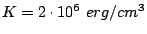and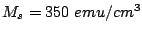. The average grain is supposed to be cylindrical in shape with radius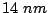and height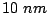. Additionally, there is a gaussian deviation of easy axis angles with standard deviation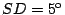and a gaussian deviation of volume centered in average volume and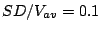. For the LLG integration we take the damping constantand the timestep(in precessional period units). Every loop is the average of three loops in order to improve statistics. Our goal is to simulate a loop with a sweep rate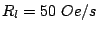and temperature.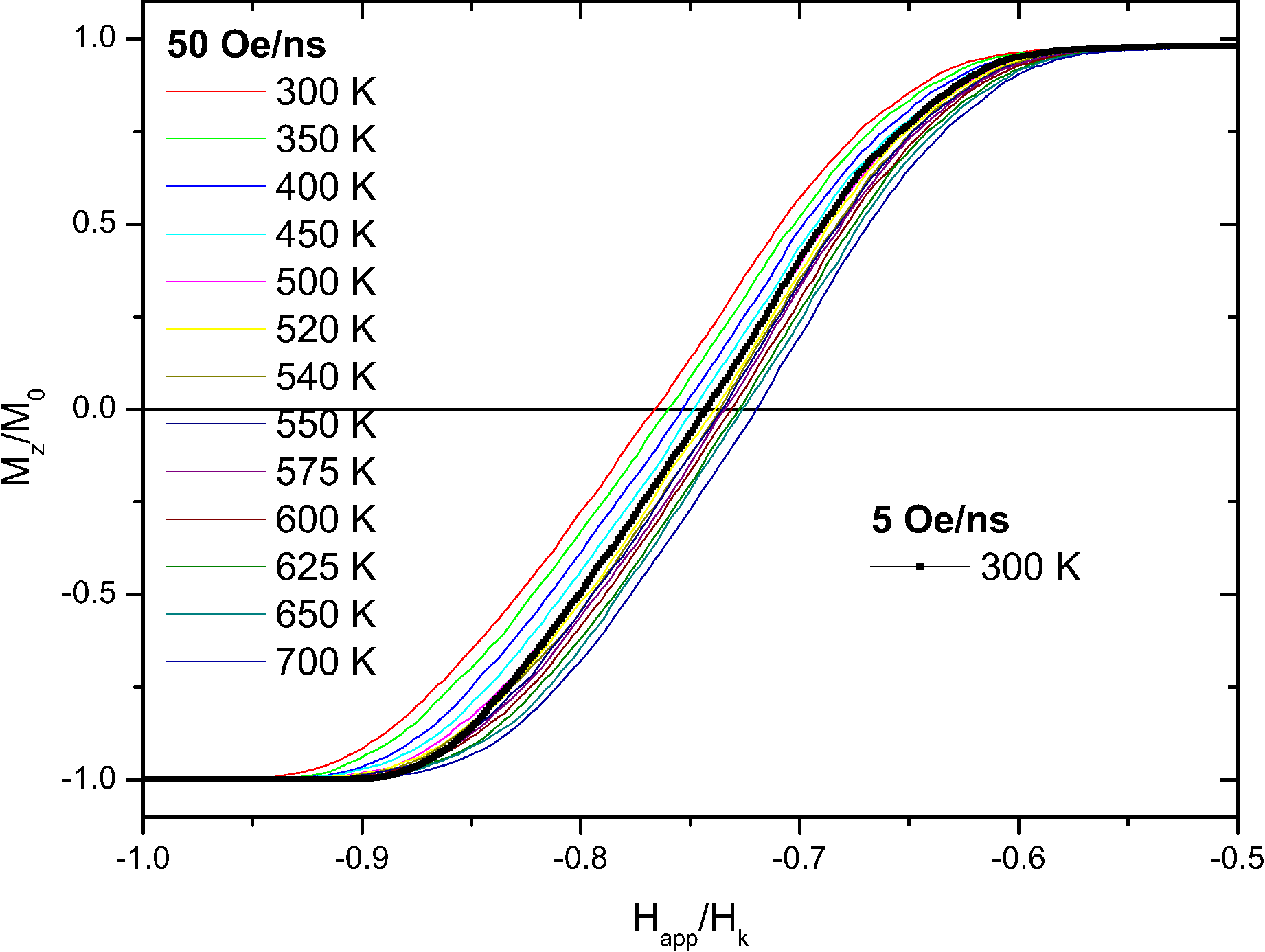First, we calculate the hysteresis loop at reference time scale. In this case we chooseand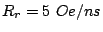. Then we look for the small time scale loopthat best matches to the previous one. In the Fig. 2.17 it is found to be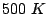loop, that is. From the Eq. (2.63) we obtain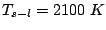. The method prediction for the loop ofandis the simulation of the loop forand.We can also try a different set of parameters to check the consistency. For example the reference time scale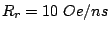and short time scale. From the Fig. 2.18 we can extract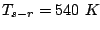and it yields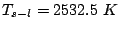.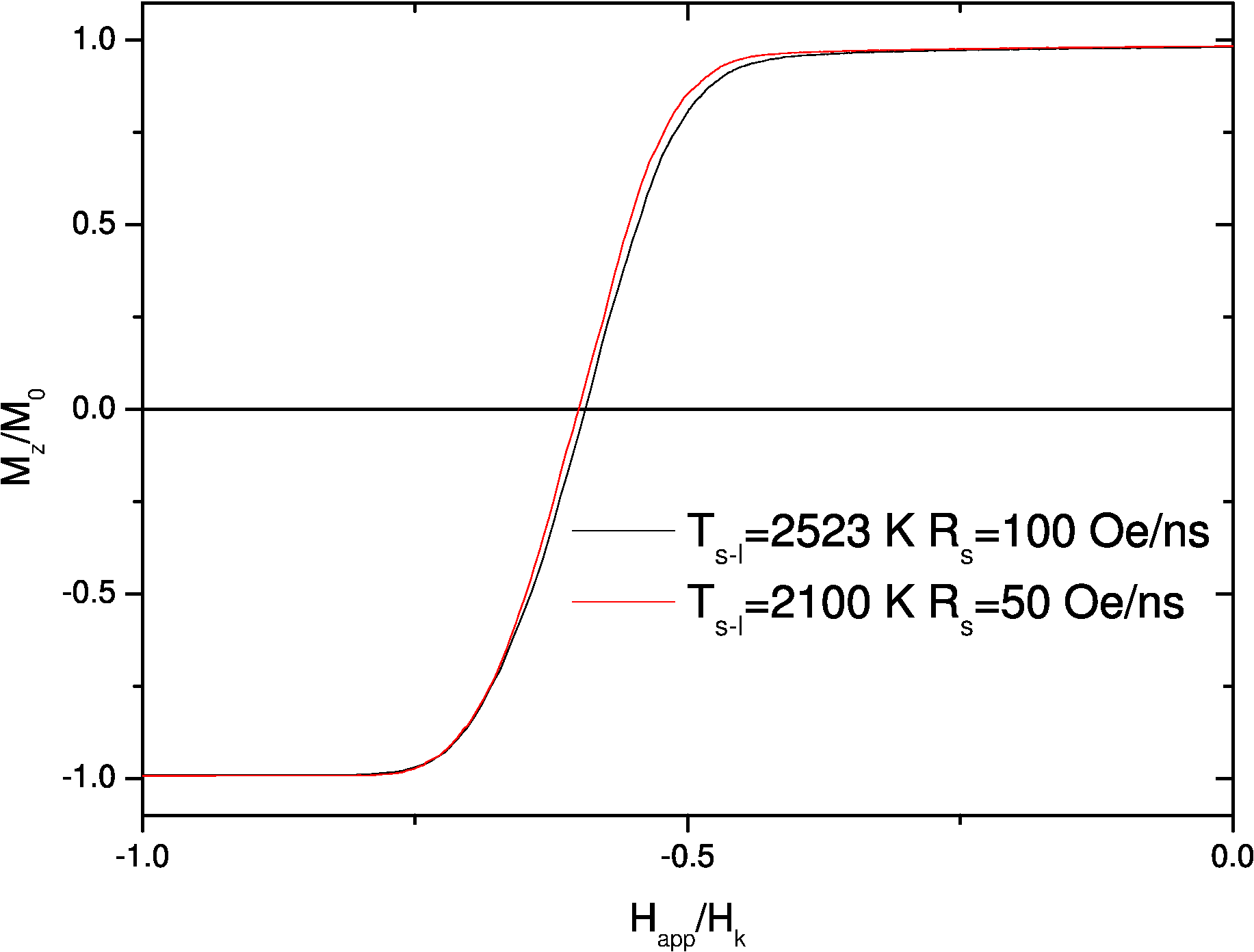The loops resulting from the application of the method are shown in Fig. 2.19. The predicted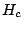value from theloops family is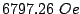and from thefamily is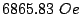. There is good agreement between the values of coercivity in both set of parameters, however, we can not check them against simulations for the aimed time scales because the resulting computational time is far from being available.

The inverse problem can be used to check the method. If we use the reference time scaleand short time scale, we can try to find the long time scalethat would match with one of the previous loops, for example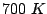. That is to say what sweep rate has. The result is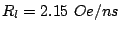and that time scale is reachable computationally. The results are shown in the Fig. 2.20.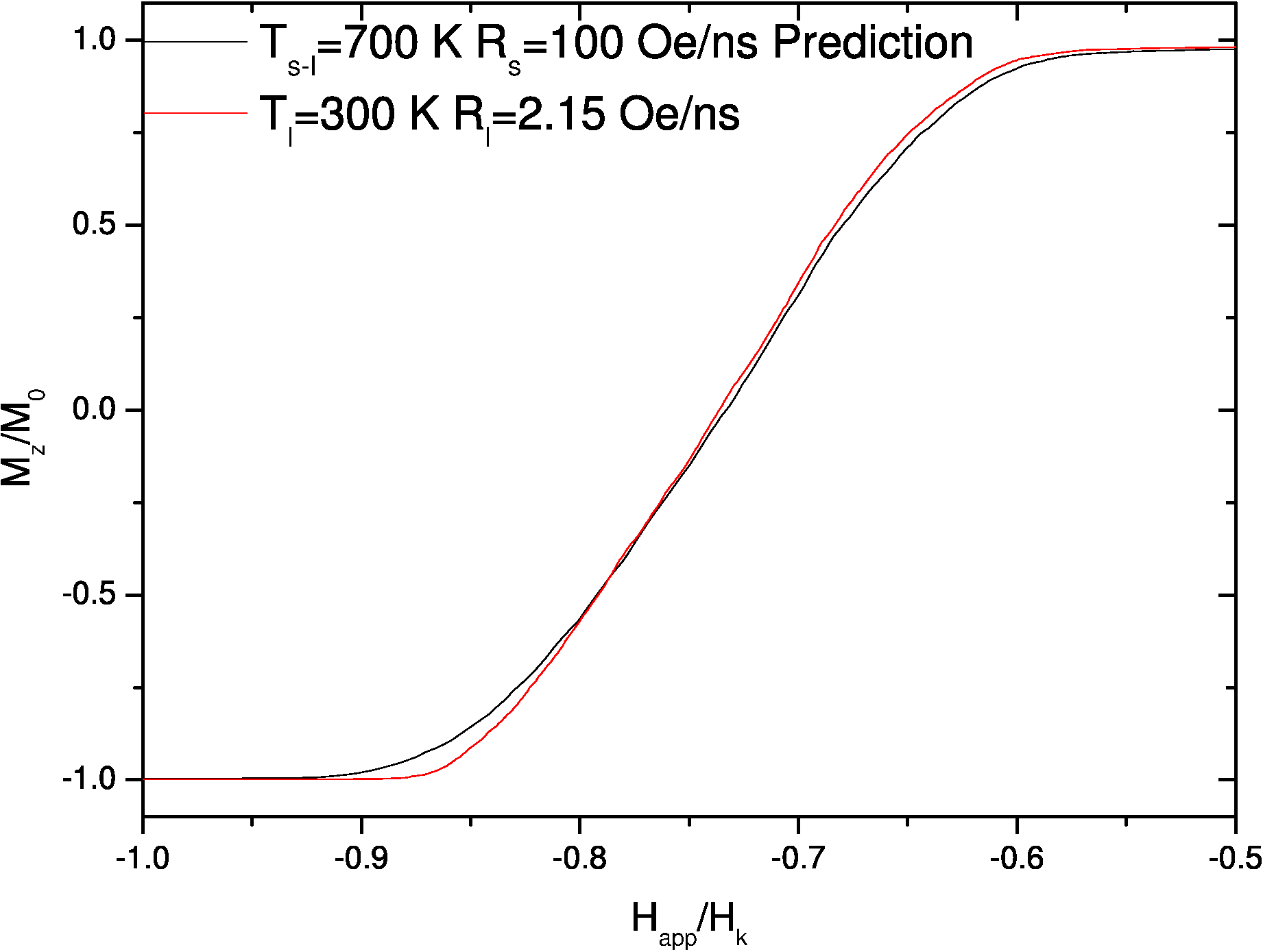The two loops should exactly match but we can see that the shapes differ specially near the saturation, although the switching fields agree reasonably. The predicted coercive field from the method isand the result from a direct LLG simulation.

The method allows to extend the reachable time scales several orders, although the method itself needs extra loops. The method has to be applied in a range of temperatures where the prefactor is constant or nearly constant. Basically, the method is a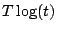scaling [Labarta 93]. Although it is possible to obtain a quantitative agreement in coercivity, the method is not good in the nucleation field and in the near negative saturation, basically because the scaling law is not valid in these regimes.

2008-04-04